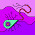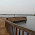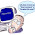## Pages

SUBSCRIBE HERE TO GET POST UPDATES VIA EMAIL :

Delivered by FeedBurner

# If Else Statement - Basic Java Tutorials For Selenium WebDriver

In my previous post, we have seen different data types in java software development language. It is very important to learn basic java tutorials If you wants to learn selenium webdriver with Java. Because If you do not have knowledge of basic java software development then you can not create single logical webdriver test case. And also you can not pass any company interview for webdriver software testing tool. So keep your eyes on these tutorials and I will take you from basic to advanced java tutorials step by step. In this post, Let me tell you the usage of If else conditional statements in java.

if, if else and nested if else statements are useful to take the decision based on conditional match.When you wants to execute some part of code if condition returns true then you need to use this kind of conditional statements.

Simple If Statement
Part of code will be executed only if specified condition returns true. If condition will return false then that code will be not executed.
Example :
``````if (i<j)
System.out.println("Value Of i("+i+") Is Smaller Than Value Of j("+j+")." );``````
In above given example, message will be printed only and only if value of variable i is less than value of variable j.

If else Statement
If condition returns true then part of if block will be executed. If condition returns false then part of else block will be executed.
Example :
``````if (i>=j)
{
System.out.println("Value Of i("+i+") Is Greater Than Or Equals To Value Of j("+j+")." );
}else
{
System.out.println("Value Of i("+i+") Is Smaller Than Value Of j("+j+")." );
}``````
In above given example, if block's message will be printed if value of variable i is greater than or equals to value of variable j. else block will be executed if value of variable i is less than value of variable j.

Nested If Else Statements
You can use nested if else statement when you wants to check multiple conditions and take decision based on it.
Example :
``````if (k<i)
{
System.out.println("Value Of k("+k+") Is Less Than Value Of i("+i+")" );
}else if (k>=i && k<=j)
{
System.out.println("Value Of k("+k+") Is In Between Value Of i("+i+") And Value Of Value Of j("+j+")" );
}else
{
System.out.println("Value Of k("+k+") Is Greater Than Value Of j("+j+")" );
}``````

In above given example, first (if) block will be executed if value of variable k is less than the value of variable i. Second (else if) block will be executed if value of variable k is greater than or equals to value of variable i and less than or equals to value of variable j. Third (else) block will be executed if value of variable k is greater than value of value of variable j. You can make a chain of if else statement if you wants to check more conditions.

This way, You can use any of above conditional statement based on your requirement. Run bellow given example in your eclipse by changing the values of variables.

``````public class IfStatements {

public static void main(String[] args) {

int i = 25;
int j = 50;
int k = 24;
//Simple If statement
System.out.println("***Simple If Statement Example***");
if (i<j) //Bellow given message will be printed only if value of variable i is less than value of variable j.
System.out.println("Value Of i("+i+") Is Smaller Than Value Of j("+j+")." );

//If Else Statement
System.out.println("");
System.out.println("***If Else Statement Example***");
if (i>=j)//Bellow given message will be printed if value of variable i is greater than or equals to value of variable j.
{
System.out.println("Value Of i("+i+") Is Greater Than Or Equals To Value Of j("+j+")." );
}else//Bellow given message will be printed if value of variable i is less than value of variable j.
{
System.out.println("Value Of i("+i+") Is Smaller Than Value Of j("+j+")." );
}

//Nested If Else Statement
System.out.println("");
System.out.println("***Nested If Else Statement Part***");
if (k<i)//Bellow given message will be printed if value of variable k is less than value of variable i.
{
System.out.println("Value Of k("+k+") Is Less Than Value Of i("+i+")" );
}else if (k>=i && k<=j)//Bellow given message will be printed if value of variable k is greater than or equals to value of variable i and less than or equals to value of variable j.
{
System.out.println("Value Of k("+k+") Is In Between Value Of i("+i+") And Value Of Value Of j("+j+")" );
}else //Bellow given message will be printed if value of variable k is greater than value of variable j.
{
System.out.println("Value Of k("+k+") Is Greater Than Value Of j("+j+")" );
}
}
}``````

Bellow given output will be printed in your eclipse console when you will run above example.
``````***Simple If Statement Example***
Value Of i(25) Is Smaller Than Value Of j(50).

***If Else Statement Example***
Value Of i(25) Is Smaller Than Value Of j(50).

***Nested If Else Statement Part***
Value Of k(24) Is Less Than Value Of i(25)``````

1.Thanks so much for this extremely helpful tutorial. It would be very nice if you would explain the nature of this syntax "+k+". Why it is necessary to wrap the "k" in pluses? Sorry in advance if that question is covered in other topics which you are referring to within this post.

2.Hi Ilya
We use + symbol to print this value in output

Now here the value of k is 24 so to print it in output we have to use +

3.+ k + means it is concatenating with the string from both sides back and front.

4.5.Nice example

6.Println function needs string to print inside the () ,whether its is a simple string of the concatenated string.In case of concatenated string all strings have to be bioperated with + sign.
e.g. in this examp,e println fuction string has 3 concatenated string.
Strin1-"Value Of i("
Strin2-") Is Smaller Than Value Of j("
Strin3-")."

And all have been concatenated with + sign.
So simple. Cheers.

7.Thank you so much..It is more helpful to all learners